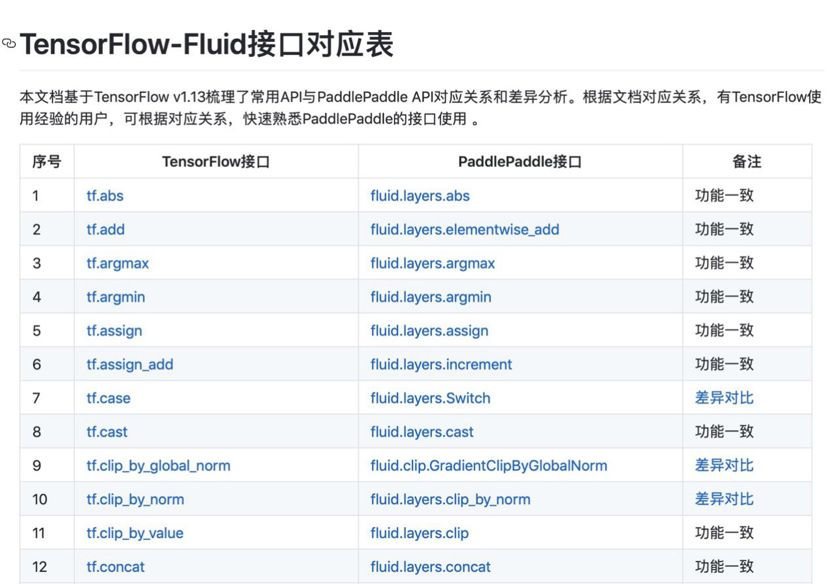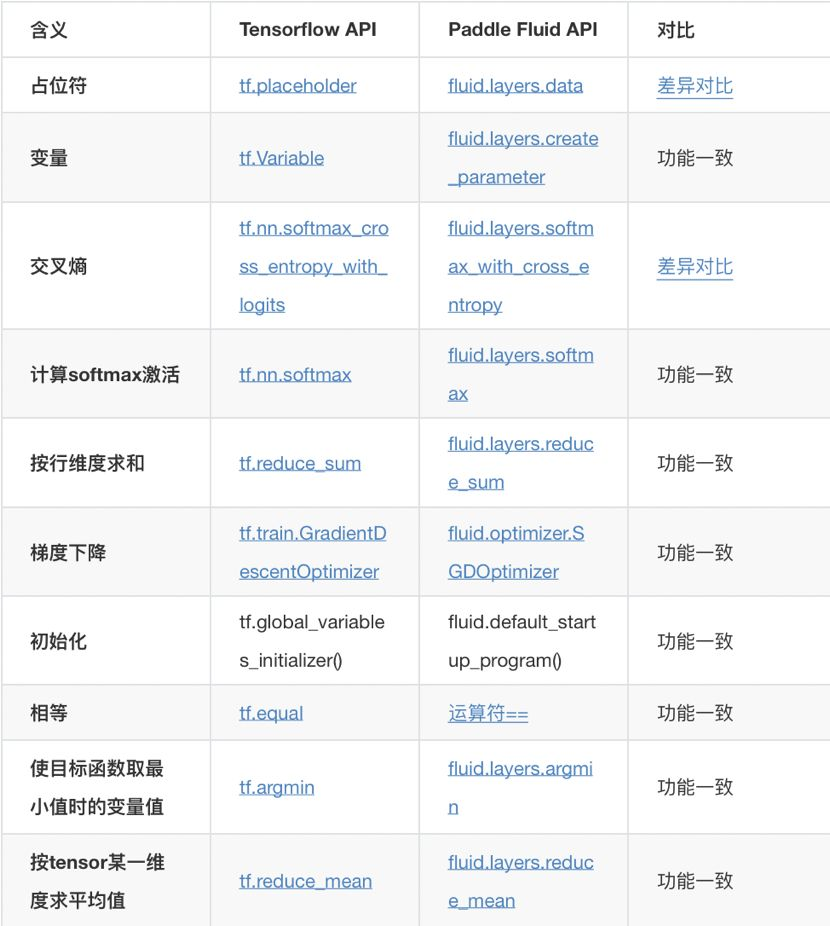1.API差异：模型的实现方式如何迁移，不同框架之间的API有没有差异？如何避免这些差异带来的模型效果的差异？

2.模型文件差异：训练好的模型文件如何迁移？转换框架后如何保证精度的损失在可接受的范围内？

3.预测方式差异：转换后的模型如何预测？预测的效果与转换前的模型差异如何？

### TensorFlow-Fluid 的API差异

``````from tensorflow.examples.tutorials.mnist import input_data
import tensorflow as tf

x = tf.placeholder(tf.float32, [None, 784])

W = tf.Variable(tf.zeros([784, 10]))
b = tf.Variable(tf.zeros())

y = tf.nn.softmax(tf.matmul(x, W) + b)

y_ = tf.placeholder("float", [None, 10])
cross_entropy = tf.reduce_sum(tf.nn.softmax_cross_entropy_with_logits(logits = y,labels = y_))

init = tf.global_variables_initializer()

sess = tf.Session()
sess.run(init)

for i in range(1, 1000):
batch_xs, batch_ys = mnist.train.next_batch(100)
sess.run(train_step, feed_dict={x: batch_xs, y_: batch_ys})

correct_prediction = tf.equal(tf.argmax(y, 1), tf.argmax(y_, 1))
accuracy = tf.reduce_mean(tf.cast(correct_prediction, 'float'))
print(sess.run(accuracy, feed_dict={x: mnist.test.images, y_: mnist.test.labels}))
``````### tf.placeholder V.S fluid.layers.data

#### 参数对比

tf.placeholder

`````` tf.placeholder(
dtype,
shape=None,
name=None
)``````

`````` paddle.fluid.layers.data(
name,
shape,
append_batch_size=True,
dtype='float32',
lod_level=0,
type=VarType.LOD_TENSOR,

• Batch维度处理

TensorFlow: 对于shape中的batch维度，需要用户使用None指定；

• 梯度是否回传

`````` layers.data(
name="data",
shape=[32, 3, 224, 224],
dtype="int64",
append_batch_size=False,
``````

### tf.nn.softmax_cross_entropy_with_logits V.S fluid.layers.softmax_with_cross_entropy

#### 参数对比

``````tf.nn.softmax_cross_entropy_with_logits(
_sentinel=None,
labels=None,
logits=None,
dim=-1,
name=None
)
``````

``````paddle.fluid.layers.softmax_with_cross_entropy(
logits,
label,
soft_label=False,
ignore_index=-100,
numeric_stable_mode=False,
return_softmax=False
)``````

TensorFlow：labels只能使用软标签，其shape为[batch, num_classes]，表示样本在各个类别上的概率分布;

TensorFlow：返回batch中各个样本的log loss；

#### 疑问点？

numeric_stable_mode：这个参数是什么呢？标志位，指明是否使用一个具有更佳数学稳定性的算法。仅在 soft_label 为 False的GPU模式下生效. 若 soft_label 为 True 或者执行场所为CPU, 算法一直具有数学稳定性。注意使用稳定算法时速度可能会变慢。默认为 True。

return_softmax: 指明是否额外返回一个softmax值， 同时返回交叉熵计算结果。默认为False。

#### 代码示例

``````data = fluid.layers.data(name='data', shape=, dtype='float32')
label = fluid.layers.data(name='label', shape=, dtype='int64')
fc = fluid.layers.fc(input=data, size=100)
out = fluid.layers.softmax_with_cross_entropy(
logits=fc, label=label)
``````

### 模型迁移

VGG_16是CV领域的一个经典模型，我以tensorflow/models下的VGG_16为例，给大家展示如何将TensorFlow训练好的模型转换为飞桨模型。

``````import urllib
import sys
def schedule(a, b, c):
per = 100.0 * a * b / c
per = int(per)
sys.stderr.flush()

fetch = urllib.urlretrieve(url, "./vgg_16.tar.gz", schedule)
``````

``````import tarfile
with tarfile.open("./vgg_16.tar.gz", "r:gz") as f:
file_names = f.getnames()
for file_name in file_names:
f.extract(file_name, "./")
``````

``````import tensorflow.contrib.slim as slim
from tensorflow.contrib.slim.nets import vgg
import tensorflow as tf
import numpy

with tf.Session() as sess:
inputs = tf.placeholder(dtype=tf.float32, shape=[None, 224, 224, 3], name="inputs")
logits, endpoint = vgg.vgg_16(inputs, num_classes=1000, is_training=False)
``````
`````` numpy.random.seed(13)
data = numpy.random.rand(5, 224, 224, 3)
input_tensor = sess.graph.get_tensor_by_name("inputs:0")
output_tensor = sess.graph.get_tensor_by_name("vgg_16/fc8/squeezed:0")
result = sess.run([output_tensor], {input_tensor:data})
numpy.save("tensorflow.npy", numpy.array(result))
``````
``````    saver = tf.train.Saver()
saver.save(sess, "./checkpoint/model")``````

TensorFlow2fluid目前支持checkpoint格式的模型或者是将网络结构和参数序列化的pb格式模型，上面下载的vgg_16.ckpt仅仅存储了模型参数，因此我们需要重新加载参数，并将网络结构和参数一起保存为checkpoint模型。

#### 将模型转换为飞桨模型

``````import tf2fluid.convert as convert
import argparse
parser = convert._get_parser()
parser.meta_file = "checkpoint/model.meta"
parser.ckpt_dir = "checkpoint"
parser.in_nodes = ["inputs"]
parser.input_shape = ["None,224,224,3"]
parser.output_nodes = ["vgg_16/fc8/squeezed"]
parser.use_cuda = "True"
parser.input_format = "NHWC"

convert.run(parser)
``````

``````INFO:root:Loading tensorflow model...
INFO:tensorflow:Restoring parameters from checkpoint/model
INFO:tensorflow:Restoring parameters from checkpoint/model
INFO:root:TotalNum:86,TraslatedNum:1,CurrentNode:inputs
INFO:root:TotalNum:86,TraslatedNum:2,CurrentNode:vgg_16/conv1/conv1_1/weights
INFO:root:TotalNum:86,TraslatedNum:3,CurrentNode:vgg_16/conv1/conv1_1/biases
INFO:root:TotalNum:86,TraslatedNum:4,CurrentNode:vgg_16/conv1/conv1_2/weights
INFO:root:TotalNum:86,TraslatedNum:5,CurrentNode:vgg_16/conv1/conv1_2/biases
...
INFO:root:TotalNum:86,TraslatedNum:10,CurrentNode:vgg_16/conv3/conv3_1/weights
INFO:root:TotalNum:86,TraslatedNum:11,CurrentNode:vgg_16/conv3/conv3_1/biases
INFO:root:TotalNum:86,TraslatedNum:12,CurrentNode:vgg_16/conv3/conv3_2/weights
INFO:root:TotalNum:86,TraslatedNum:13,CurrentNode:vgg_16/conv3/conv3_2/biases

INFO:root:TotalNum:86,TraslatedNum:86,CurrentNode:vgg_16/fc8/squeezed
INFO:root:Model translated!
``````

#### 预测结果差异

``````import numpy

numpy.random.seed(13)
data = numpy.random.rand(5, 224, 224, 3).astype("float32")
# NHWC -> NCHW
data = numpy.transpose(data, (0, 3, 1, 2))

results = model.inference(feed_dict={model.inputs:data})

``````

#### 对比模型损失

``````import numpy
print(numpy.max(diff))
``````

#### 打印输出

``````6.67572e-06
``````

1. 转换后的模型需要注意输入格式，飞桨中输入格式需为NCHW格式。

此例中不涉及到输入中间层，如卷积层的输出，需要了解的是飞桨中的卷积层输出，卷积核的shape与TensorFlow有差异。

2. 模型转换完后，检查转换前后模型的diff，需要测试得到的最大diff是否满足转换需求。

### 参考资料：### 【H.264】SPS 计算帧率方法_sps可以获取帧率么_等风来不如迎风去的博客-程序员宅基地

FFmpeg 帧率要从解码器获取才是正途FFmpeg(三) 编解码相关函数理解_weixin_33966095的博客-程序员信息网static double r2d(AVRational r){ return r.num==0||r.den == 0 ? 0 :(double)r.num/(double)r.den;} fps = r2d(as->avg_frame_rate);#include <jni.h>#include <stri._sps可以获取帧率么

### Oracle11g安装教程，附安装包下载地址。_oracle11g安装包-程序员宅基地

​本篇主要介绍oracle的安装过程及安装中需要注意的东西，后附有安装包链接及如何下载安装包。针对开头的安装流程的链接补充了一些东西，主要可参考下方链接，其中要注意本篇所补充的。主要流程可以参考这个：Oracle 11g服务器安装详细步骤——图文教程_Resourceful!的博客-程序员宅基地_oracle11g安装教程完整版重点重点重点！！！不要只看上述链接，上述链接可以下载成功，但有些设置没有直接设置好，后期修改着特别麻烦，最好在下载的过程中直接设置好。以下补充上述链接过程中的一些设置问题._oracle11g安装包

### item_search_shop_sellerid - 根据sellerid获得店铺的所有商品_Onebound_Ed的博客-程序员宅基地

item_search_shop_sellerid - 根据sellerid获得店铺的所有商品测试网址：点击注册测试Result Object:---------------------------------------{ "seller_id": "1746960915", "page": "1", "has_next_page": "true", "real_total_results": "8189", "total_results": "8189", "page_size":

### Hadoop ERROR tool.ImportTool: Encountered IOException running import-程序员宅基地

ERROR tool.ImportTool: Encountered IOException running import[training@training hadoop]\$ lscustomers.java orders.java sqoop_details.sh suppliers.javaemployees.java products.java sqoop.sh[tr..._error tool.importtool: encountered ioexception running import job: org.apach